## Content

### Finding domains and ranges

The natural domain of a function is the set of all allowable input values. We will call it the domain of the function $$f$$, denoted by $$\mathrm{domain}(f)$$. The range of the function $$f$$ is the set of all possible output values: $\mathrm{range}(f) = \{\, f(x) : x\in\mathrm{domain}(f) \,\}.$

For a particular function $$f(x)$$, determining $$\mathrm{domain}(f)$$ and $$\mathrm{range}(f)$$ is sometimes quite complicated.

#### Example

What is the domain and range of the function $$f(x)=4-x^2$$?

#### Solution

Here a graph of the function helps.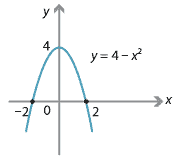Since $$f(x)$$ is defined for all real numbers, we have $$\mathrm{domain}(f) = \mathbb{R}$$. We can see from the graph that $$\mathrm{range}(f) = \{\, y : y\leq 4 \,\} = (-\infty,4]$$.

#### Example

What is the domain and range of $$f(x)=-\dfrac{7}{x}$$?

#### Solution

The graph of $$y=f(x)$$ is a rectangular hyperbola.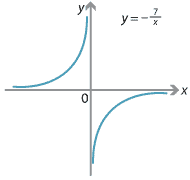From the graph, we can see that \begin{align*} \mathrm{domain}(f) &= \{\, x\in\mathbb{R} \mid x\neq 0 \,\} = \mathbb{R}\setminus \{0\} \\ \mathrm{range}(f) &= \{\, y\in\mathbb{R} \mid y\neq 0 \,\} = \mathbb{R}\setminus \{0\}. \end{align*}

#### Example

What is the domain and range of $$f(x)=7^x-5$$?

#### Solution

This is a vertical translation of an exponential function.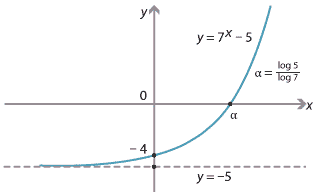Hence, $$\mathrm{domain}(f) = \mathbb{R}$$ and $$\mathrm{range}(f) = (-5,\infty)$$.

#### Example

What is the domain and range of $$f(x)=\sqrt{16-x^2}$$?

#### Solution

We have \begin{align*} y = \sqrt{16-x^2} \ &\iff\ y^2 = 16-x^2 \hspace{4mm}\text{and}\hspace{4mm} y \geq 0 \\ &\iff\ x^2+y^2=16 \hspace{4mm}\text{and}\hspace{4mm} y \geq 0. \end{align*}

So the graph of $$f(x)$$ is the top half of the circle with centre the origin and radius 4.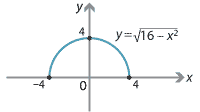Hence, $$\mathrm{domain}(f) = [-4,4]$$ and $$\mathrm{range}(f) = [0,4]$$.

In the next example, we find the domain and range without first drawing the graph.

#### Example

Find the domain and range of $$f(x)=\sqrt{1-2x}$$.

#### Solution

We first find the domain of $$f(x)$$:

\begin{align*} f(x) \text{ is defined} \ &\iff\ 1-2x \geq 0 \\ &\iff\ x \leq \tfrac{1}{2}. \end{align*}

So $$\mathrm{domain}(f) = (-\infty,\dfrac{1}{2}]$$.

Every non-negative real number can be obtained as a value of $$f(x) = \sqrt{1-2x}$$. Thus $$\mathrm{range}(f) = [0,\infty)$$.

We can sketch the graph of this function by noticing that

\begin{align*} y = \sqrt{1-2x} \ &\iff\ y^2 = 1-2x \hspace{4mm}\text{and}\hspace{4mm} y \geq 0 \\ &\iff\ x = \tfrac{1}{2}(1 - y^2) \hspace{4mm}\text{and}\hspace{4mm} y \geq 0. \end{align*}

So this is 'half' of a rotated and translated parabola.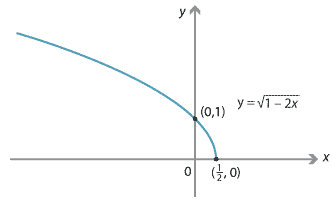##### Exercise 3
Find the domain and range of
1. $$f(x) = \sqrt{(x-1)(x-2)}$$
2. $$g(x) = \sqrt{1-2\sin2x}$$.

#### Example

Find the domain and range of $$f(x)=x^{\frac{1}{3}}$$. Sketch the graph of $$y=f(x)$$.

#### Solution

Clearly $$\mathrm{domain}(f) = \mathbb{R}$$, since all real numbers have a cube root. As $$x\to \infty$$, $$f(x)\to\infty$$ and as $$x\to -\infty$$, $$f(x)\to -\infty$$. Since $$f$$ is continuous, it follows that $$\mathrm{range}(f) = \mathbb{R}$$.

We have $$y=x^{\frac{1}{3}}$$ if and only if $$x=y^3$$. The graph of $$y=x^3$$ is as follows.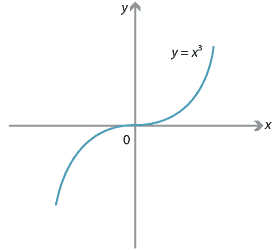The graph of $$x=y^3$$, or $$y=x^\frac{1}{3}$$, is obtained by reflecting in the line $$y = x$$.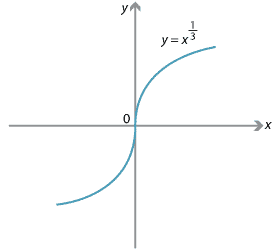##### Exercise 4

What is the domain and range of $$f(x)=3\tan 2x$$? Sketch the graph of $$y=3\tan 2x$$.

#### Example

Find the domain and range of $$f(x)=|x|$$, where as usual

$|x| = \begin{cases} -x &\text{if } x < 0, \\ x &\text{if } x \geq 0. \end{cases}$

#### Solution

By drawing the graph of $$y=|x|$$, we see that $$\mathrm{domain}(f) = \mathbb{R}$$ and $$\mathrm{range}(f) = [0,\infty)$$.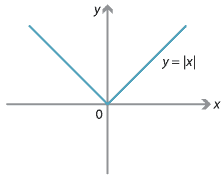##### Exercise 5

Let $$\lfloor x\rfloor$$ denote the largest integer less than or equal to $$x$$. So, for example, $$\lfloor \pi \rfloor=3$$.

1. Let $$f(x) = \lfloor x \rfloor$$. Sketch the graph of $$y=f(x)$$. Find $$\mathrm{domain}(f)$$ and $$\mathrm{range}(f)$$.
2. Let $$g(x) = x - \lfloor x \rfloor$$. Sketch the graph of $$y=g(x)$$. Find $$\mathrm{domain}(g)$$ and $$\mathrm{range}(g)$$.

(In some books, the notation for $$\lfloor x\rfloor$$ is $$[x]$$.)

Next page - Links forward - Functions between sets